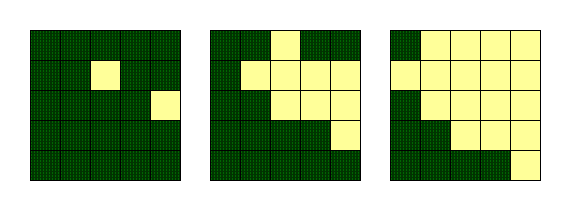### Problem Statement

Desertification (the process of good land turning into desert) is a severe problem on Bob's island. Bob's island is a rectangular grid of cells. You are given a String[] island that shows the current state of Bob's island. The j-th character of the i-th element of island is 'D' if cell in row i, column j of the grid is desert and is 'F' if this cell is forest.

The desert spreads each year as follows:
• If a cell is desert, it remains desert forever.
• If a cell is forest and it is adjacent to at least one desert cell (in one of the four orthogonal directions), it becomes desert after one year.
• Otherwise the cell remains forest for another year.
Return the number of desert cells after T years.

### Definition

 Class: Desertification Method: desertArea Parameters: String[], int Returns: int Method signature: int desertArea(String[] island, int T) (be sure your method is public)

### Constraints

-island will contain between 1 and 10 elements, inclusive.
-Each element of island will contain between 1 and 10 characters, inclusive.
-Each character in island will be 'D' or 'F'.
-Each element of island will contain the same number of characters.
-T will be between 1 and 1,000,000,000, inclusive.

### Examples

0)

 ```{"FFF", "FDF", "FFF"}``` `1`
`Returns: 5`
 After one year, the island will be: ```FDF DDD FDF ```
1)

 ```{"FFF", "FDF", "FFF"}``` `2`
`Returns: 9`
 All cells will be desert after two years.
2)

 ```{"FFFFF", "FFDFF", "FFFFD", "FFFFF", "FFFFF"}``` `2`
`Returns: 17`
 In this example, the picture on the left represents the initial state for Bob's island. After two years, the island state will become the picture on the right (dark green represents forest and pale yellow represents desert). Thus, the number of desert cells after 2 years is 17.3)

 ```{"FFFFFF", "FFFFFF", "FFFFFF", "FFFFFF"}``` `1000000000`
`Returns: 0`
4)

 ```{"FFFFFDFFFF", "FDFDFFFFFF", "FFFFFFFFFD", "FFFFFFFFFF", "DDFFFFFFFF", "FFFFFFFFFD", "FFFFFFFFFF", "FFFFFFFDFF", "FFFFFFFDFF", "FFFFDDFFFF"}``` `3`
`Returns: 90`
5)

 ```{"FFFFFDFFFF", "FDFDFFFFFF", "FFFFFFFFFD", "FFFFFFFFFF", "DDFFFFFFFF", "FFFFFFFFFD", "FFFFFFFFFF", "FFFFFFFDFF", "FFFFFFFDFF", "FFFFDDFFFF"}``` `98765432`
`Returns: 100`

#### Problem url:

http://www.topcoder.com/stat?c=problem_statement&pm=10725

#### Problem stats url:

http://www.topcoder.com/tc?module=ProblemDetail&rd=14180&pm=10725

rng_58

#### Testers:

Rustyoldman , timmac , ivan_metelsky , mohamedafattah , it4.kp

#### Problem categories:

Simple Search, Iteration